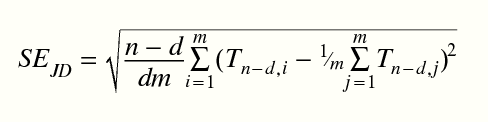# Sib-pair Command: set jackknife

 Class Global Name set jackknife Arguments | off

Controls the number of deleted observations used by the describe command's algorithm for the estimation of standard errors for familial correlations for a quantitative trait. If off modifier chosen, reverts to default behaviour.

The standard error formula is:JSE=((n-d)/dm * SUM(ri-mean(ri, i=1, m))2)1/2

where d is the number of observations dropped for each pseudosample,
ri is the test statistic value for the ith pseudosample,
m is the number of pseudosamples,
and n is the number of observations [Shao and Tu 1995].

The default behaviour is for this value to be calculated as required as:

max(1, min(10, Nobs/10)).

Example:

```>> set jackknife
NOTE:  Jackknife is adaptive (delete-1 to delete-10).
>> set jackknife 10
NOTE:  Jackknife is delete-10.

```

 << (set emit) Up to index >> (set batch)